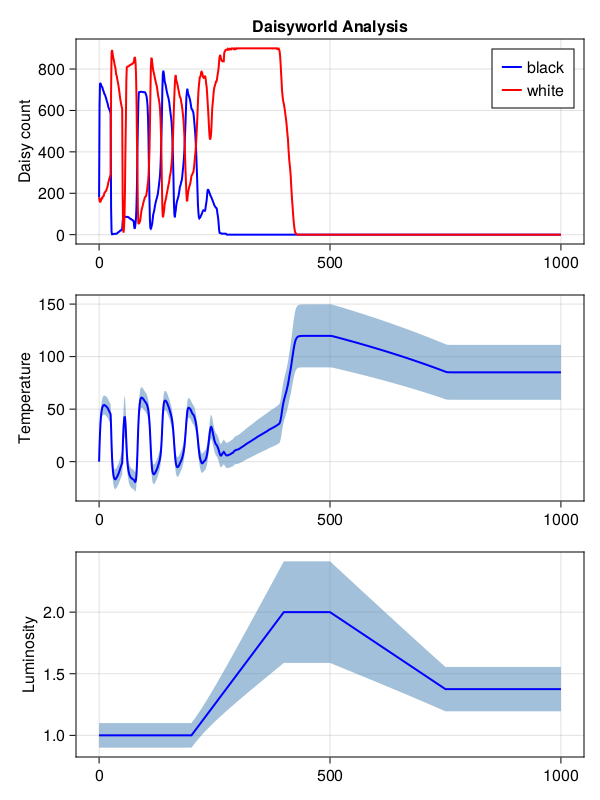# Providing uncertainty with Measurements.jl

Measurements.jl provides automatic error propagation, and integrates seamlessly with much of the Julia ecosystem.

Here, we'll slightly modify the Daisyworld example, to simulate some measurement uncertainty in our world's parameters.

## Setup

First we'll construct our agents.

using Agents
using Measurements

mutable struct Daisy <: AbstractAgent
id::Int
pos::Dims{2}
breed::Symbol
age::Int
albedo::AbstractFloat # Allow Measurements
end

mutable struct Land <: AbstractAgent
id::Int
pos::Dims{2}
temperature::AbstractFloat # Allow Measurements
end

Notice that there is only one small difference between this version and the original example model: the use of AbstractFloat instead of Float64 for the albedo and temperature parameters. Behaviour between these two types is practically equivalent from our perspective, but it allows us to use an uncertain value for our two parameters. 1.0 ± 0.1 rather than 1.0 for example. We could also be specific here and bind the parameters with type Measurement{Float64} as well.

Next, we'll implement all the important functions for DaisyWorld. If you want to know what each of these functions do, see the Daisyworld example, as they are copied directly from there.

using CairoMakie
using Statistics: mean
import DrWatson: @dict
import StatsBase

const DaisyWorld = ABM{<:GridSpace,Union{Daisy,Land}}

function update_surface_temperature!(pos::Dims{2}, model::DaisyWorld)
ids = ids_in_position(pos, model)
absorbed_luminosity = if length(ids) == 1
(1 - model.surface_albedo) * model.solar_luminosity
else
(1 - model[ids].albedo) * model.solar_luminosity
end
local_heating = absorbed_luminosity > 0 ? 72 * log(absorbed_luminosity) + 80 : 80
T0 = model[ids].temperature
model[ids].temperature = (T0 + local_heating) / 2
end

function diffuse_temperature!(pos::Dims{2}, model::DaisyWorld)
ratio = get(model.properties, :ratio, 0.5)
ids = nearby_ids(pos, model)
meantemp = sum(model[i].temperature for i in ids if model[i] isa Land) / 8
land = model[ids_in_position(pos, model)]
land.temperature = (1 - ratio) * land.temperature + ratio * meantemp
end

function propagate!(pos::Dims{2}, model::DaisyWorld)
ids = ids_in_position(pos, model)
if length(ids) > 1
daisy = model[ids]
temperature = model[ids].temperature
seed_threshold = (0.1457 * temperature - 0.0032 * temperature^2) - 0.6443
if rand(model.rng) < seed_threshold
empty_neighbors = Tuple{Int,Int}[]
neighbors = nearby_positions(pos, model)
for n in neighbors
if length(ids_in_position(n, model)) == 1
push!(empty_neighbors, n)
end
end
if !isempty(empty_neighbors)
seeding_place = rand(model.rng, empty_neighbors)
a = Daisy(nextid(model), seeding_place, daisy.breed, 0, daisy.albedo)
end
end
end
end

function agent_step!(agent::Daisy, model::DaisyWorld)
agent.age += 1
agent.age >= model.max_age && kill_agent!(agent, model)
end

agent_step!(agent::Land, model::DaisyWorld) = nothing

function model_step!(model)
for p in positions(model)
update_surface_temperature!(p, model)
diffuse_temperature!(p, model)
propagate!(p, model)
end
model.tick += 1
solar_activity!(model)
end

function solar_activity!(model::DaisyWorld)
if model.scenario == :ramp
if model.tick > 200 && model.tick <= 400
model.solar_luminosity += model.solar_change
end
if model.tick > 500 && model.tick <= 750
model.solar_luminosity -= model.solar_change / 2
end
elseif model.scenario == :change
model.solar_luminosity += model.solar_change
end
end
solar_activity! (generic function with 1 method)

Now, we can write a constructor function, and use uncertainly values which will propagate automatically through our model.

function daisyworld(;
griddims = (30, 30),
max_age = 25,
init_white = 0.2,
init_black = 0.2,
albedo_white = 0.75,
albedo_black = 0.25,
# Surface albedo measurements are complicated for our satellites perhaps
surface_albedo = 0.4 ± 0.15,
# Measurements from the sun are generally stable, but fluctuate around 10%
solar_change = 0.005 ± 0.002,
solar_luminosity = 1.0 ± 0.1,
scenario = :default,
)

space = GridSpace(griddims)
properties = @dict max_age surface_albedo solar_luminosity solar_change scenario
properties[:tick] = 0
daisysched(model) = [a.id for a in allagents(model) if a isa Daisy]
model = ABM(
Union{Daisy,Land},
space;
scheduler = daisysched,
properties = properties,
warn = false,
)

# An uncertain initial temperature, solely for type stability
fill_space!(Land, model, 0.0 ± 0.0)
grid = collect(positions(model))
num_positions = prod(griddims)
white_positions =
StatsBase.sample(grid, Int(init_white * num_positions); replace = false)
for wp in white_positions
wd = Daisy(nextid(model), wp, :white, rand(model.rng, 0:max_age), albedo_white)
end
allowed = setdiff(grid, white_positions)
black_positions =
StatsBase.sample(allowed, Int(init_black * num_positions); replace = false)
for bp in black_positions
wd = Daisy(nextid(model), bp, :black, rand(model.rng, 0:max_age), albedo_black)
end

return model
end
daisyworld (generic function with 1 method)

You see we've included uncertainty in four places: surface albedo and initial temperature, and the two solar luminosity values. We do not require changes to any model code, nor handle these parameters in any special way; for example 2.0 * surface_albedo is a regular operation. Errors will be propagated under the hood automatically.

## Visualizing the Result

Similar to the Daisyworld example, we will now check out how the surface temperature and daisy count fares when solar luminosity ramps up.

First, some helper functions

black(a) = a.breed == :black
white(a) = a.breed == :white
daisies(a) = a isa Daisy

land(a) = a isa Land
adata = [(black, count, daisies), (white, count, daisies), (:temperature, mean, land)]

mdata = [:solar_luminosity]
1-element Vector{Symbol}:
:solar_luminosity

And now the simulation

model = daisyworld(scenario = :ramp)
agent_df, model_df =

f = Figure(resolution = (600, 800))
ax = f[1, 1] = Axis(f, ylabel = "Daisy count", title = "Daisyworld Analysis")
lb = lines!(ax, agent_df.step, agent_df.count_black_daisies, linewidth = 2, color = :blue)
lw = lines!(ax, agent_df.step, agent_df.count_white_daisies, linewidth = 2, color = :red)
leg =
f[1, 1] = Legend(
f,
[lb, lw],
["black", "white"],
tellheight = false,
tellwidth = false,
halign = :right,
valign = :top,
margin = (10, 10, 10, 10),
)

ax2 = f[2, 1] = Axis(f, ylabel = "Temperature")
highband =
lowband =
band!(ax2, agent_df.step, lowband, highband, color = (:steelblue, 0.5))
lines!(
ax2,
agent_df.step,
linewidth = 2,
color = :blue,
)

ax3 = f[3, 1] = Axis(f, ylabel = "Luminosity")
highband =
Measurements.value.(model_df.solar_luminosity) +
Measurements.uncertainty.(model_df.solar_luminosity)
lowband =
Measurements.value.(model_df.solar_luminosity) -
Measurements.uncertainty.(model_df.solar_luminosity)
band!(ax3, agent_df.step, lowband, highband, color = (:steelblue, 0.5))
lines!(
ax3,
agent_df.step,
Measurements.value.(model_df.solar_luminosity),
linewidth = 2,
color = :blue,
)
f